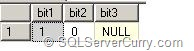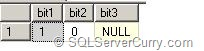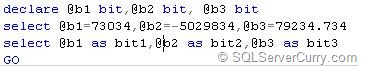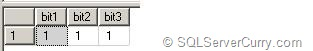### SQL Server Bit Data Type and how it Stores Values

SQL Server supports the bit datatype which can be used to store flag values like 'true' or 'false'. However it should be noted how the values are stored in the bit column. This article gives you an overview of the same.

Consider this example

declare @b1 bit,@b2 bit, @b3 bit
select @b1=1,@b2=0,@b3=null
select @b1 as bit1,@b2 as bit2,@b3 as bit3
GOThe result shows 1,0 and null.

You can also use the string values 'true' and 'false' in place of 1 and 0.

declare @b1 bit,@b2 bit, @b3 bit
select @b1='true',@b2='false',@b3=null
select @b1 as bit1,@b2 as bit2,@b3 as bit3
GOThe result shows 1,0 and null.

However other than the string values 'true' and 'false', the bit datatype will not accept any other string as shown below:

declare @b1 bit
select @b1='test'
select @b1

The above query will throw the following error:

Msg 245, Level 16, State 1, Line 3
Conversion failed when converting the varchar value 'test' to data type bit.

You can also assign any number to the bit value, but it will convert it to 1,0 or null. Remember that a bit datatype can only store these three values.

The following example shows that how numbers other than 0 or 1 are converted by the bit datatypeOUTPUTAs the result shows, any value which is not null or 0 will be converted to 1.